# Sum of First N natural Numbers in C++

## Sum of First N Natural Numbers in C++:

In this article, I am going to discuss the Sum of First N Natural Numbers in C++ with Examples. Please read our previous article where we discussed the Area of Triangle Program in C++ with Examples.

##### Sum of First N Natural Numbers in C++:

In this article let us write a program for finding the sum of first natural numbers using C++ language. Already we have seen the formula in our previous article. So let us write a complete program. let us see what does it mean by the sum of the first n natural number:

1 + 2 + 3 + … + n.

Natural numbers start from one onwards. If we want to find the sum of all these numbers, let’s say the sum of n natural numbers, the formula is:So, for example if I want to find out: 1 + 2 + 3 + 4 + 5. Then applying the formula as: 5 * 6 / 2 = 15. This is coming to 15, so the sum is 15. This is a well-known formula and we already have explained it in our previous article. Next, we want to write a program for the sum of n terms. Let us first write down a flowchart.

##### Flowchart for Sum of First N Natural Numbers

So, for the flow chart, we should follow these steps: input, process, and output. What is input for this program? ‘n’ is input. And what is the output? ‘sum’ is output. And ‘n (n+1) / 2’ is the formula for processing. So let us draw the flow chart.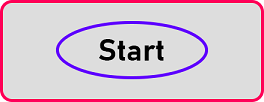This is the starting point of the flowchart. In the first step, we should take the input so we should have an input box and here I will print “Enter n”. We should know the value of n. We have to read the value of n.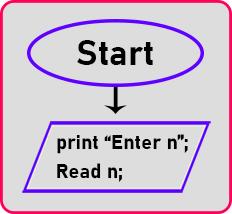So, so enter the value of n and store it in the variable ‘n’. Here the input is over. The next step is a process. So, what’s the processing, calculating the sum, so how to calculate sum, sum assigned with the formula ‘n * (n+1) / 2’: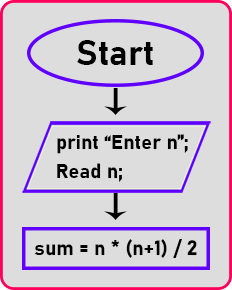This is processing. The next step is output. So, we have the result. Where is the result? In variable ‘sum’ so print that sum. So, we will write here and we will give a message that print ‘sum is‘ then after this we will write the variable name.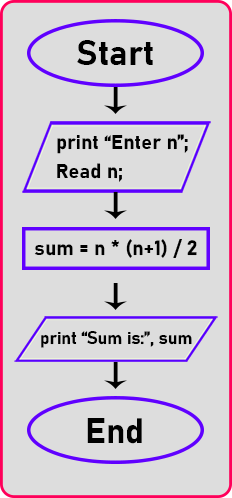Then after this end of flow chart. So, this is the flow chart for finding the sum of the first n natural number using this formula. Now next thing is the algorithm:

##### Algorithm for Sum of First N Natural Numbers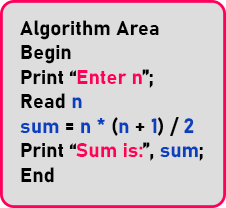##### Sum of First N Natural Numbers Program Code in C++ Language:

Now we will write a C++ program for finding the sum of n natural numbers:

```#include <iostream>
using namespace std;
int main()
{
int n, sum;
cout << "Enter n: ";
cin >> n;
sum = n * (n + 1) / 2;
cout << "Sum is: " << sum;
return 0;
}
```
###### Output: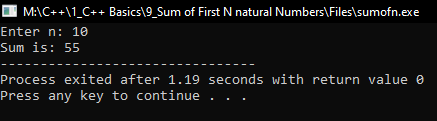In the next article, I am going to discuss the Roots of Quadratic Equations in C++ with Examples. Here, in this article, I try to explain the Sum of First N Natural Numbers in C++ with Examples and I hope you enjoy this Sum of First N Natural Numbers in C++ with Examples article.